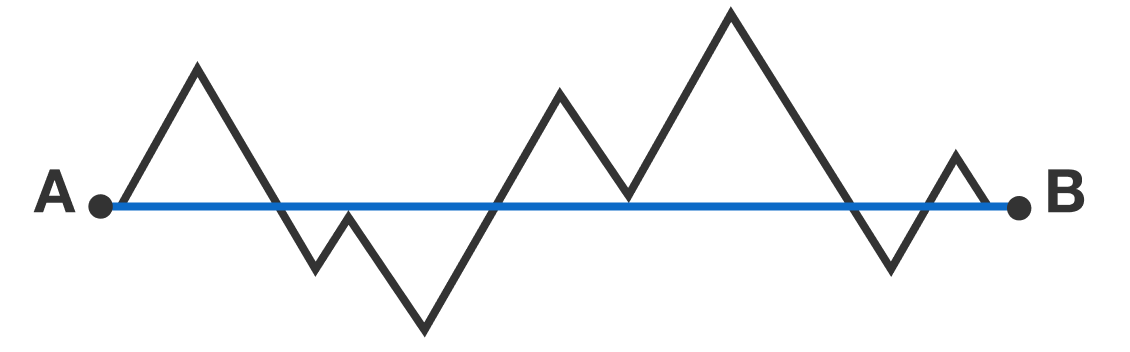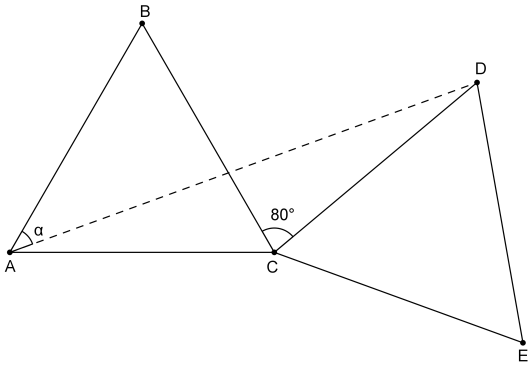Geometry

# Classification of Triangles: Level 1 ChallengesArjit drew an isosceles right angled triangle on the board. What is the measure of the base angle?The polygonal path connecting points A and B forms 7 equilateral triangles.

If the distance between point A to point B is 20 units, then how long is the distance of the path (in units)?

Let $\triangle ABC$ and $\triangle CDE$ be equilateral triangles of the same size, and $\angle BCD=80^\circ$ between them. Find the measure of $\angle BAD$ in degrees.Which non-degenerate triangle cannot have one of its angles as ${91}^\circ$?

Which non-degenerate triangle can have one of its angles as ${89}^{\circ}$?

×